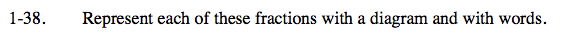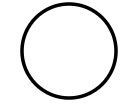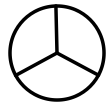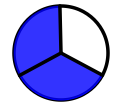### Home > MC2 > Chapter 1 > Lesson 1.1.5 > Problem1-38

1-38.

Represent each of these fractions with a diagram and with words.

1. $\frac { 2 } { 3 }$

2. $1 \frac { 1 } { 8 }$

3. $\frac { 6 } { 9 }$$\text{a}. \; \frac{2}{3}$

The denominator (below the fraction bar) shows how many sections there are in the diagram. The numerator (above the fraction bar) shows how many of those sections are filled in.

Draw a shape.

Divide the shape into equal sections. Since the denominator is 3, divide the shape into 3 sections of equal sizes.

Since the numerator is 2, fill in 2 of those 3 sections you created.$\text{b}. \; 1\frac{1}{8}$

Read the number aloud. One AND one eighth means that you have one whole diagram and one eighth of another. Now try drawing your own diagram.

$\text{c}. \; \frac{6}{9}$

Refer to part (a) for help.